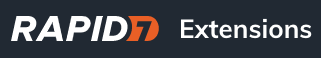# A selection of Network Flow queries for alerting on inbound applications

ENTA addon is needed for these queries. They cover applications which are often targeted during inbound attacks.

Inbound RDP
Network traffic where direction is INBOUND and application protocol is RDP (remote desktop protocol)
`where(app_protocol="rdp" direction="INBOUND")`

Inbound MSSQL
Network traffic where direction is INBOUND and application protocol is Microsoft SQL
`where(app_protocol="msql" direction="INBOUND")`

Inbound Telnet
Network traffic where direction is INBOUND and application protocol is Telnet
`where(app_protocol="telnet" direction="INBOUND")`

Inbound SMB
Network traffic where direction is INBOUND and application protocol is SMB
`where(app_protocol="smb" direction="INBOUND")`

Inbound SSH
Network traffic where direction is INBOUND and application protocol is SSH
`where(app_protocol="ssh" direction="INBOUND")`

While HTTP and HTTPS can be common inbound applications, it may be worth monitoring for their presence on sensitive or critical networks.

Inbound HTTP
Network traffic where direction is INBOUND and application protocol is HTTP
`where(app_protocol="http" direction="INBOUND")`

Inbound HTTPS
Network traffic where direction is INBOUND and application protocol is HTTPS
`where(app_protocol="https" direction="INBOUND")`

4 Likes